Courses

# Test: 35 Year JEE Previous Year Questions: Units & Measurements

## 20 Questions MCQ Test Physics For JEE | Test: 35 Year JEE Previous Year Questions: Units & Measurements

Description
This mock test of Test: 35 Year JEE Previous Year Questions: Units & Measurements for JEE helps you for every JEE entrance exam. This contains 20 Multiple Choice Questions for JEE Test: 35 Year JEE Previous Year Questions: Units & Measurements (mcq) to study with solutions a complete question bank. The solved questions answers in this Test: 35 Year JEE Previous Year Questions: Units & Measurements quiz give you a good mix of easy questions and tough questions. JEE students definitely take this Test: 35 Year JEE Previous Year Questions: Units & Measurements exercise for a better result in the exam. You can find other Test: 35 Year JEE Previous Year Questions: Units & Measurements extra questions, long questions & short questions for JEE on EduRev as well by searching above.
QUESTION: 1

### A dense collection of equal number of electrons and positive ions is called neutral plasma. Certain solids containing fixed positive ions surrounded by free electrons can be treated as neutral plasma. Let ‘N’ be the number density of free electrons, each of mass ‘m’. When the electrons are subjected to an electric field, they are displaced relatively away from the heavy positive ions. If the electric field becomes zero, the electrons begin to oscillate about the positive ions with a natural angular frequency ‘ωp’ which is called the plasma frequency. To sustain the oscillations, a time varying electric field needs to be applied that has an angular frequency ω, where a part of the energy is absorbed and a part of it is reflected. As ω approaches ωp all the free electrons are set to resonance together and all the energy is reflected. This is the explanation of high reflectivity of metals. Q. Taking the electronic charge as ‘e’ and the permittivity as ‘ε0’. Use dimensional analysis to determine the correct expression for ωp.

Solution: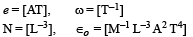We do not want Ampere [A] in the expression. This is only possible when ∈0 occurs as square. Therefore options a and b are incorrect.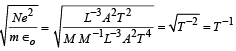QUESTION: 2

### A dense collection of equal number of electrons and positive ions is called neutral plasma. Certain solids containing fixed positive ions surrounded by free electrons can be treated as neutral plasma. Let ‘N’ be the number density of free electrons, each of mass ‘m’. When the electrons are subjected to an electric field, they are displaced relatively away from the heavy positive ions. If the electric field becomes zero, the electrons begin to oscillate about the positive ions with a natural angular frequency ‘ωp’ which is called the plasma frequency. To sustain the oscillations, a time varying electric field needs to be applied that has an angular frequency ω, where a part of the energy is absorbed and a part of it is reflected. As ω approaches ωp all the free electrons are set to resonance together and all the energy is reflected. This is the explanation of high reflectivity of metals. Q. Estimate the wavelength at which plasma reflection will occur for a metal having the density of electrons N ≈ 4 x 1027 m–3. Taking ε0 = 10–11 and mass m ≈ 10–30, ≈ where these quantities are in proper SI units.

Solution: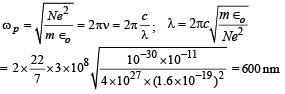QUESTION: 3

### Identify the pair whose dimensions are equal

Solution: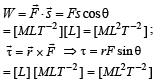QUESTION: 4

Dimen sion of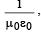where symbols have their usual meaning, are

Solution:

We know that the velocity of light in vacuum is given by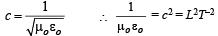QUESTION: 5

The physical quantities not having same dimensions are

Solution:

Momentum = mv = [MLT–1]

Planck’s constant,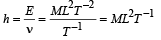QUESTION: 6

Which one of the following represents the correct dimensions of the coefficient of viscosity?

Solution:

From stokes law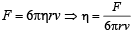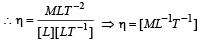QUESTION: 7

Out of the following pair , which one does NOT have identical dimensions is

Solution:

Moment of Inertia, I = Mr2

[I] = [ML2]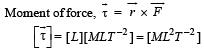QUESTION: 8

The dimension of magnetic field in M, L, T and C (coulomb) is given as

Solution:

We know that F = q v B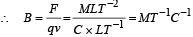QUESTION: 9

A body of mass m = 3.513 kg is moving along the x-axis with a speed of 5.00 ms–1. The magnitude of its momentum is recorded as

Solution:

Momentum, p = m × v = (3.513) × (5.00) = 17.565 kg m/s
= 17.6 (Rounding off to get three significant figures)

QUESTION: 10

Two full turns of the circular scale of a screw gauge cover a distance of 1mm on its main scale. The total number of divisions on the circular scale is 50. Further, it is found that the screw gauge has a zero error of – 0.03 mm. While measuring the diameter of a thin wire,  a student notes the main scale reading of 3 mm and the number of circular scale divisions in line with the main scale as 35. The diameter of the wire is

Solution:

Least count of screw gauge = 0.5/50 mm 0.01mm

reading × L.C] – (zero error)
= [3 + 35 × 0.01] – (–0.03) = 3.38 mm

QUESTION: 11

In an experiment the angles are required to be measured using an instrument, 29 divisions of the main scale exactly coincide with the 30 divisions of the vernier scale. If the smallest division of the main scale is half- a degree (= 0.5°), then the least count of the instrument is :

Solution:

30 Divisions of vernier scale coincide with 29 divisions of main scales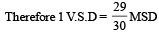Least count = 1 MSD – 1VSD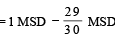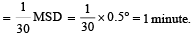QUESTION: 12

The respective number of significant figures for the numbers 23.023, 0.0003 and 2.1 × 10–3 are

Solution:

Number of significant figures in 23.023 = 5
Number of significant figures in 0.0003 = 1
Number of significant figures in 2.1 × 10–3 = 2

QUESTION: 13

A screw gauge gives the following reading when used to measure the diameter of a wire.
Main scale reading : 0 mm
Circular scale reading : 52 divisions
Given that 1mm on main scale corresponds to 100 divisions of the circular scale. The diameter of wire from the above data is:

Solution: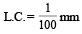Diameter of wire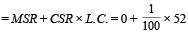= 0.52 mm = 0.052 cm

QUESTION: 14

Resistance of a given wire is obtained by measuring the current flowing in it and the voltage difference applied across it. If the percentage errors in the measurement of the current and the voltage difference are 3% each, then error in the value of resistance of the wire is :

Solution: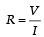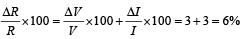QUESTION: 15

A spectrometer gives the following reading when used to measure the angle of a prism.
Main scale reading : 58.5 degree
Vernier scale reading : 09 divisions
Given that 1 division on main scale corresponds to 0.5 degree.
Total divisions on the Vernier scale is 30 and match with 29 divisions of the main scale. The angle of the prism from the above data :

Solution:

Vernier scale reading = 09 division
least count of Vernier = 0.5°/30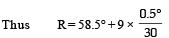R = 58.65

QUESTION: 16

Let [ε0] denote the dimensional formula of the permittivity of vacuum. If M = mass, L = length, T = time and A = electric current, then

Solution:

Using Coulomb law,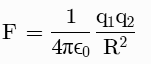Hence,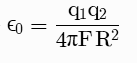Substituting the units in the equation: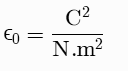=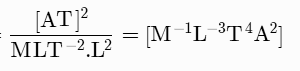QUESTION: 17

A student measured the length of a rod and wrote it as 3.50 cm. Which instrument did he use to measure it?

Solution:

Measured length of rod = 3.50 cm
For vernier scale with 1 Main Scale Division = 1 mm
9 Main Scale Division = 10 Vernier Scale Division,
Least count = 1 MSD –1 VSD = 0.1 mm

QUESTION: 18

The period of oscillation of a simple pendulum is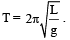Measured value of L is 20.0 cm known to 1 mm accuracy and time for 100 oscillations of the pendulum is found to be 90 s using a wrist watch of 1s resolution. The accuracy in the determination of g is :

Solution: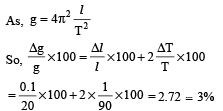QUESTION: 19

A student measures the time period of 100 oscillations of a simple pendulum four times. The data set is 90s, 91s, 92s and 95s. If the minimum division in the measuring clock is ls, then the reported mean time should be

Solution:

Sum of all observation= 90+91+95+92=368

Average =368/4=92

Sum of modulus errors=2+1+3=6

Average error =6/4=1.5,rounded of 2.

Final answer =(92 ± 2) s

QUESTION: 20

A screw gauge with a pitch of 0.5 mm and a circular scale with 50 divisions is used to measure the thickness of a thin sheet of Aluminium. Before starting the measurement, it is found that wen the two jaws of the screw gauge are brought in contact, the 45th division coincides with the main scale line and the zero of the main scale is barely visible. What is the thickness of the sheet if the main scale reading is 0.5 mm and the 25th division coincides with the main scale line?

Solution: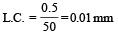Zero error = 5 × 0.01 = 0.05 mm (Negative)
Reading = (0.5 + 25 × 0.01) + 0.05 = 0.80 mm